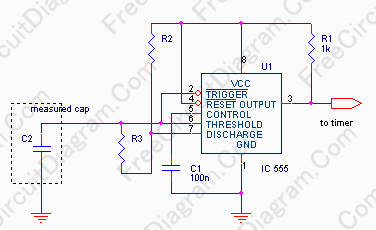# Capacitance Meter Using 555 OscillatorThe frequency formula of 555 oscillator is popularly known. For a known resistors and capacitor, the frequency can be determined by a formula. From the math logic, if the frequency and the resistors are known, then an unknown capacitor could be determined by the inverse of the formula. Anyone who has a frequency counter are able to use the following circuit to determine the capacity of an unknown capacitor.The formula for the period of this circuit is T=0.69(R2+2R3)C2. The resistors R2 and R3 are fixed, so the period will be proportional to the unknown C2. The period can be measured by standard timer-counter available in your lab. You can select R2 and R3 to measure pico farad, nano farad, and micro farad.

There is a little problem when measuring electrolytic capacitor around 1uF. Form about 1 second measurement, the would be higher enough to make the current leakage of the capacitor cause invalid reading. In this case, reading of 1000uF can be better than 1uF, because if we set for 1000uF = 1 second the the needed resistors could be much lower than the capacitors leakage resistance. The following example show some cases:

• For R2=1k and R3=220R would give a 1000uF capacitor a one second period reading
• For R2=1M and R3=220k would give a 1uF (non electrolytic) a one second period reading

For the mechanical construction, make sure that the wiring is stable to give a fixed wiring capacitance, so we can compensate this fix wiring capacitance addition by subtracting the reading with the floating (no capacitor) reading, especially when you use for measuring small capacitors.